# 跳跃表数据结构与算法分析

1. 通过搜索引用，可以保证从任意元素开始，搜索到在列表中间隔为k的元素的任意期望时间是O(logk)
2. 实现线性表的常规操作（例如_将元素插入到列表第k个元素后面_）

# 预览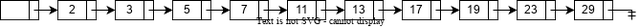Figure.2表示一个链表，在该链表中，每个一个节点就有一个附加的指针指向它在表中的前两个位置上的节点。正因为这个前向指针，在最坏情况下最多考查⌈N/2⌉+1个节点。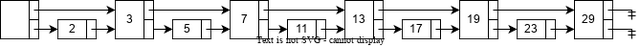Figure.2 Linked List with fingers to the 2nd forward elements

Figure.3将这种想法扩展，每个序数是4的倍数的节点都有一个指针指向下一个序数为4的倍数的节点。只有⌈N/4⌉+2个节点被考查。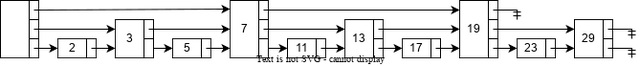Figure.3 Linked List with fingers to the 4th forward elements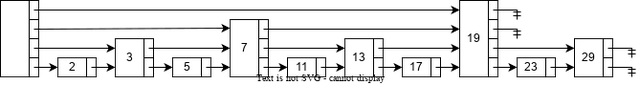Figure.4 Linked List with fingers to the 2ith forward elements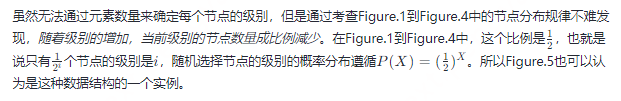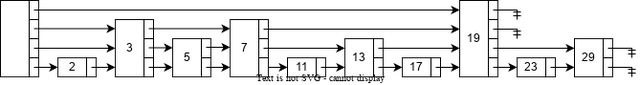Figure.5 Skip List

# 数据结构

1. 所有元素在跳跃表中都是由一个节点表示。
2. 每个节点都有一个高度或级别，有时候也可以称之为阶（step），节点的级别是一个与元素总数无关的随机数。规定NULL的级别是∞。
3. 每个级别为k的节点都有k个前向指针，且第i个前向指针指向下一个级别为i或更高的节点。
4. 每个节点的级别都不会超过一个明确的常量MaxLevel。整个跳跃表的级别是所有节点的级别的最高值。如果跳跃表是空的，那么跳跃表的级别就是1。

``````#define SKIP_LIST_KEY_TYPE     int
#define SKIP_LIST_VALUE_TYPE   int
#define SKIP_LIST_MAX_LEVEL    32
#define SKIP_LIST_P            0.5

struct Node {
SKIP_LIST_KEY_TYPE    key;
SKIP_LIST_VALUE_TYPE  value;
struct Node          *forwards[]; // flexible array member
};

struct SkipList {
int          level;
};

struct Node *CreateNode(int level) {
struct Node *node;
assert(level > 0);
node = malloc(sizeof(struct Node) + sizeof(struct Node *) * level);
return node;
}

struct SkipList *CreateSkipList() {
struct SkipList *list;
int              i;

list = malloc(sizeof(struct SkipList));
for (i = 0; i < SKIP_LIST_MAX_LEVEL; i++) {
}
list->level = 1;

return list;
}

``````

# 算法

## 搜索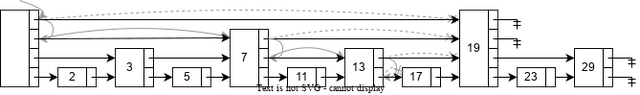Figure.6 A search path to find element 17 in Skip List

``````struct Node *SkipListSearch(struct SkipList *list, SKIP_LIST_KEY_TYPE target) {
struct Node *current;
int          i;

for (i = list->level - 1; i >= 0; i--) {
while (current->forwards[i] && current->forwards[i]->key < target) {
current = current->forwards[i];
}
}

current = current->forwards;
if (current->key == target) {
return current;
} else {
return NULL;
}
}

``````

## 插入和删除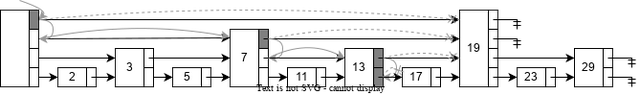Figure.7 Search path for inserting element 16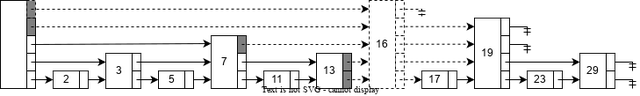Figure.8 Insert element 16 and adjust forward pointers

``````struct Node *SkipListInsert(struct SkipList *list, SKIP_LIST_KEY_TYPE key, SKIP_LIST_VALUE_TYPE value) {
struct Node *update[SKIP_LIST_MAX_LEVEL];
struct Node *current;
int          i;
int          level;

for (i = list->level - 1; i >= 0; i--) {
while (current->forwards[i] && current->forwards[i]->key < target) {
current = current->forwards[i];
}
update[i] = current;
}

current = current->forwards;
if (current->key == target) {
current->value = value;
return current;
}

level = SkipListRandomLevel();
if (level > list->level) {
for (i = list->level; i < level; i++) {
}
}

current = CreateNode(level);
current->key = key;
current->value = value;

for (i = 0; i < level; i++) {
current->forwards[i] = update[i]->forwards[i];
update[i]->forwards[i] = current;
}

return current;
}

``````

Figure.9和Figure.10展示了在跳跃表中删除元素19的过程。首先，在Figure.9中执行与搜索相同的查询过程，在每个级别遍历到的最后一个元素在对应层级的前向指针被标记为灰色，表示稍后将会对齐进行调整。接下来在Figure.10中，首先通过调整前向指针将元素19对应的节点从跳跃表中卸载，因为元素19对应的节点是级别最高的节点，因此将其从跳跃表中移除后需要调整跳跃表的级别。Figure.10中所有虚线部分都表示调整后的效果。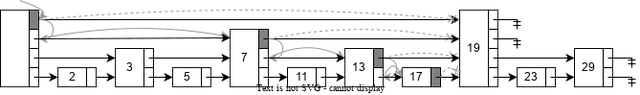Figure.9 Search path for deleting element 19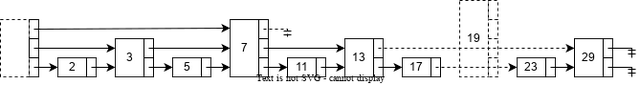Figure.10 Delete element 19 and adjust forward pointers

``````struct Node *SkipListDelete(struct SkipList *list, SKIP_LIST_KEY_TYPE key) {
struct Node *update[SKIP_LIST_MAX_LEVEL];
struct Node *current;
int          i;

for (i = list->level - 1; i >= 0; i--) {
while (current->forwards[i] && current->forwards[i]->key < key) {
current = current->forwards[i];
}
update[i] = current;
}

current = current->forwards;
if (current && current->key == key) {
for (i = 0; i < list->level; i++) {
if (update[i]->forwards[i] == current) {
update[i]->forwards[i] = current->forwards[i];
} else {
break;
}
}

while (list->level > 1 && list->head->forwards[list->level - 1] == NULL) {
list->level--;
}
}

return current;
}

``````

## 生成随机级别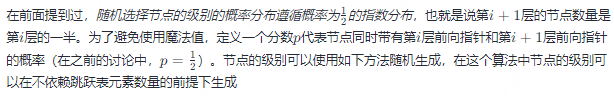``````int SkipListRandomLevel() {
int level;
level = 1;
while (random() < RAND_MAX * SKIP_LIST_P && level <= SKIP_LIST_MAX_LEVEL) {
level++;
}
return level;
}

``````

1 2 3 4 5 6 7 8
2147540777 1073690199 536842769 268443025 134218607 67116853 33563644 16774262
9 10 11 12 13 14 15 16
8387857 4193114 2098160 1049903 523316 262056 131455 65943
17 18 19 20 21 22 23 24
32611 16396 8227 4053 2046 1036 492 249
25 26 27 28 29 30 31 32
121 55 34 16 7 9 2 1

## MaxLevel的选择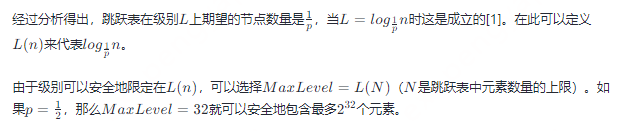# 分析

## 空间复杂度分析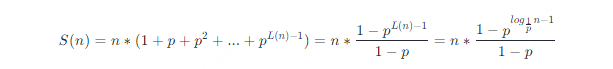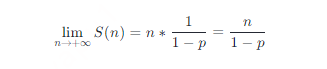## 时间复杂度分析

### 非形式化分析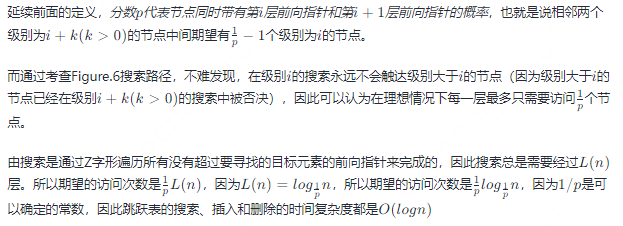### 形式化分析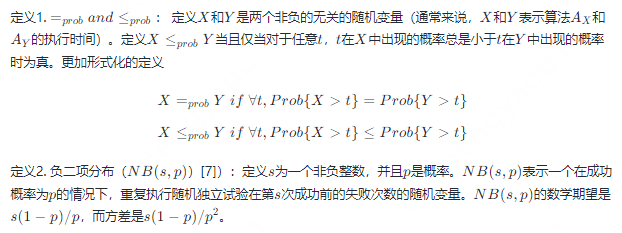C(n)≤probC=prob(L(n)−1)+NB(L(n)−1,p)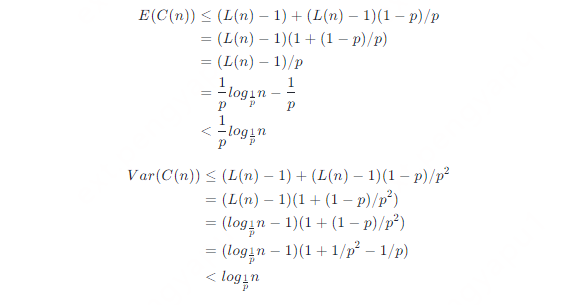### P的选择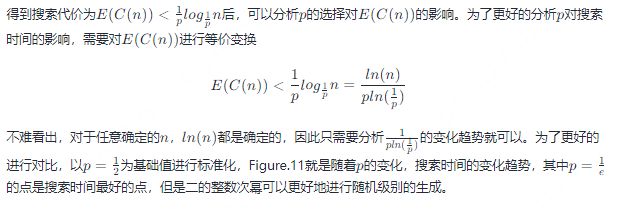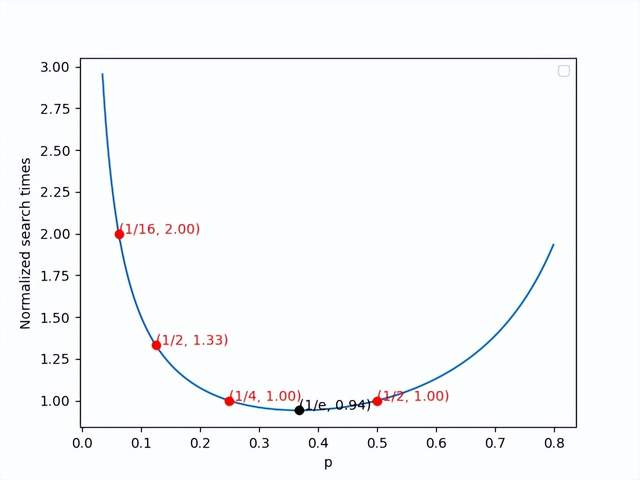Figure.11 Normalized search times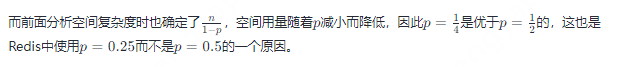# 扩展

## 快速随机访问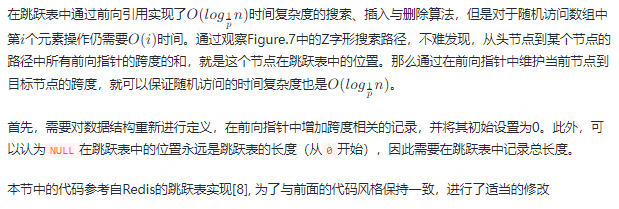``````#define SKIP_LIST_KEY_TYPE     int
#define SKIP_LIST_VALUE_TYPE   int
#define SKIP_LIST_MAX_LEVEL    32
#define SKIP_LIST_P            0.5

struct Node; // forward definition

struct Forward {
struct Node *forward;
int          span;
}

struct Node {
SKIP_LIST_KEY_TYPE    key;
SKIP_LIST_VALUE_TYPE  value;
struct Forward        forwards[]; // flexible array member
};

struct SkipList {
int          level;
int          length;
};

struct Node *CreateNode(int level) {
struct Node *node;
assert(level > 0);
node = malloc(sizeof(struct Node) + sizeof(struct Forward) * level);
return node;
}

struct SkipList *CreateSkipList() {
struct SkipList *list;
int              i;

list = malloc(sizeof(struct SkipList));
for (i = 0; i < SKIP_LIST_MAX_LEVEL; i++) {
}

list->level = 1;

return list;
}

``````

``````struct Node *SkipListInsert(struct SkipList *list, SKIP_LIST_KEY_TYPE key, SKIP_LIST_VALUE_TYPE value) {
struct Node *update[SKIP_LIST_MAX_LEVEL];
struct Node *current;
int          indices[SKIP_LIST_MAX_LEVEL];
int          i;
int          level;

for (i = list->level - 1; i >= 0; i--) {
if (i == list->level - 1) {
indices[i] = 0;
} else {
indices[i] = indices[i + 1];
}

while (current->forwards[i].forward && current->forwards[i].forward->key < target) {
indices[i] += current->forwards[i].span;
current = current->forwards[i].forward;
}
update[i] = current;
}

current = current->forwards.forward;
if (current->key == target) {
current->value = value;
return current;
}

level = SkipListRandomLevel();
if (level > list->level) {
for (i = list->level; i < level; i++) {
indices[i] = 0;
update[i]->forwards[i].span = list->length;
}
}

current = CreateNode(level);
current->key = key;
current->value = value;

for (i = 0; i < level; i++) {
current->forwards[i].forward = update[i]->forwards[i].forward;
update[i]->forwards[i].forward = current;
current->forwards[i].span = update[i]->forwards[i].span - (indices - indices[i]);
update[i]->forwards[i].span = (indices - indices[i]) + 1;
}

list.length++;

return current;
}

``````
``````struct Node *SkipListDelete(struct SkipList *list, SKIP_LIST_KEY_TYPE key) {
struct Node *update[SKIP_LIST_MAX_LEVEL];
struct Node *current;
int          i;

for (i = list->level - 1; i >= 0; i--) {
while (current->forwards[i].forward && current->forwards[i].forward->key < key) {
current = current->forwards[i].forward;
}
update[i] = current;
}

current = current->forwards.forward;
if (current && current->key == key) {
for (i = 0; i < list->level; i++) {
if (update[i]->forwards[i].forward == current) {
update[i]->forwards[i].forward = current->forwards[i];
update[i]->forwards[i].span += current->forwards[i].span - 1;
} else {
break;
}
}

while (list->level > 1 && list->head->forwards[list->level - 1] == NULL) {
list->level--;
}
}

return current;
}

``````

# 参考文献

1. Pugh, W. (1989). A skip list cookbook. Tech. Rep. CS-TR-2286.1, Dept. of Computer Science, Univ. of Maryland, College Park, MD [July 1989]
2. Pugh, W. (1989). Skip lists: A probabilistic alternative to balanced trees. Lecture Notes in Computer Science, 437–449. https://doi.org/10.1007/3-540-51542-9_36
3. Weiss, M. A. (1996).Data Structures and Algorithm Analysis in C (2nd Edition)(2nd ed.). Pearson.
4. Aragon, Cecilia & Seidel, Raimund. (1989). Randomized Search Trees. 540-545. 10.1109/SFCS.1989.63531.
5. Wikipedia contributors. (2022b, November 22).Finger search. Wikipedia. https://en.wikipedia.org/wiki/Finger_search